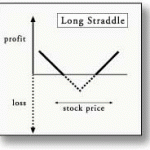April 16, 2012A Long Straddle is created by buying both a put and a call with the same terms.  In other words, you buy both a put and a call on the same underlying stock, with the same strike price, and the same expiration date.

A straddle purchase allows the buyer to make big potential profits if the stock moves far enough in either direction.  The buyer has a predetermined maximum loss which is equal to the amount of their initial investment.

In practice, you would usually establish a Long Straddle on a relatively volatile stock.  One that has the potential to move far enough up or down to make the straddle profitable in the established timeframe.

This strategy is especially useful when option premiums are low.  Low premiums allow the option buyer to establish a long straddle at a lower cost.

Now, a large percentage of Long Straddles will produce losses when held to expiration.  But there is a very small probability of losing your entire investment when using a Long Straddle.

Here’s an example of how a Long Straddle works:

Let’s assume we’re going to establish a Long Straddle position on Qualcomm (QCOM), which is trading at \$50 per share.  Further assume we buy the QCOM July 50 call for \$3 and the QCOM July 50 put for \$2.

The cost to establish this long straddle is \$5 (the sum of the premiums paid for each option).  The investment required to purchase the straddle is the net debit.

The breakeven points at expiration are simple to calculate.

For the call option, you add the sum of the two option premiums (\$5) to the call option’s strike price of \$50 to get \$55.  For the put option, you merely subtract the sum of the two option premiums (\$5) from the put option strike price of \$50 to get \$45.

So, the option buyer would lose money if QCOM is trading between those two breakeven points at expiration.  And he would make potentially huge profits if QCOM moved a great distance away from \$50 in either direction.

More specifically…

If QCOM is trading at \$50 when the options expire, the buyer would lose his entire investment.  This makes sense as both options would expire worthless.

But if the stock were above \$55 at expiration, the call portion of the straddle would be worth more than 5 points.  In other words, the buyer would make money on the trade even though his put option expired worthless.

The same would be true if QCOM was trading for less than \$45 at expiration.  In that scenario, the put would be worth more than 5 points and the buyer would have a profit even though the call option expired worthless.